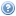# Course List for 2018The undergraduate courses on this list are also available in a timetable view.

Course Title Trimester(s) Teaching Home
ENGR 122 Engineering Mathematics with Calculus 2
ENGR 123 Engineering Mathematics with Logic and Statistics 2
MATH 132 Intr to Mathematical Thinking 1, 3
MATH 211 Foundations of Algebra, Analysis and Topology 1
MATH 301 Differential Equations 2
MATH 309 Mathematical Logic 2
MATH 311 Algebra 2
MATH 324 Coding and Cryptography 2
MATH 335 Computability and Complexity 1
MATH 377 Probability and Random Processes 1
MATH 433 Model Theory 2
MATH 434 Set Theory 1
MATH 435 Computability and Complexity 2
MATH 436 Galois Theory and Number Theory 1
MATH 441 Measure Theory 1
MATH 442 Functional Analysis 1
MATH 453 Lie Groups and Lie Algebras 2
MATH 461 Differential Equations 1
MATH 477 Probability 1
MATH 482 Special Topic: Topics in Machine Learning 2
MATH 483 Special Topic: Operator Algebra 2
SCIE 201 Special Topic: Data Management, Programming and Applications 1 Home Page
STAT 193 Statistics in Practice 1, 2, 3
STAT 332 Statistical Inference 2